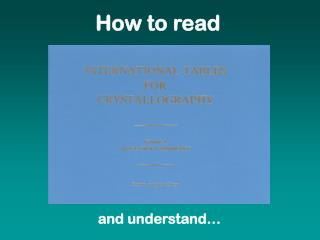DownloadDownload PresentationTitle

# Title

Télécharger la présentation## Title

- - - - - - - - - - - - - - - - - - - - - - - - - - - E N D - - - - - - - - - - - - - - - - - - - - - - - - - - -
##### Presentation Transcript

1. How to read Title and understand…

2. Page

3. Left system crystal system

4. Left point group point group symbol

5. Left space group1 space group symbol international (Hermann-Mauguin) notation

6. Left space group2 space group symbol Schönflies notation

7. Left symmetry diagram diagram of symmetry operations positions of symmetry operations

8. Left positions diagram diagram of equivalent positions

9. Left origin origin position vs. symmetry elements

10. Left asymmetric unit definition of asymmetric unit (not unique)

11. Left Patterson Patterson symmetry Patterson symmetry group is always primitive centrosymmetric without translational symmetry operations

12. Right positions equivalent positions

13. Right special positions special positions

14. Right subgroups subgroups

15. Right absences systematic absences systematic absences result from translational symmetry elements

16. Right generators group generators

17. Individual items Interpretation of individual items

18. Left system crystal system

19. Systems 7 (6) Crystal systems Triclinic a ¹ b ¹ c a, b, g ¹ 90º Monoclinic a ¹ b ¹ c a=g = 90º, b ¹ 90º Orthorhombic a ¹ b ¹ c a=b=g = 90º Tetragonal a = b ¹ c a=b=g = 90º Rhombohedral a = b = c a=b=g Hexagonal a = b ¹ c a=b= 90º , g = 120º Cubic a = b = c a=b=g = 90º

20. Left point group point group symbol

21. Point groups describe symmetry of finite objects (at least one point invariant) Point groups Set of symmetry operations: rotations and rotoinversions (or proper and improper rotations) mirror = 2-fold rotation + inversion Combination of two symmetry operations gives another operation of the point group (principle of group theory)

22. Point groups describe symmetry of finite objects (at least one point invariant) Point groups general _ _ _ _ _ _ _ _ _ _ _ __ Schönflies International Examples Cn N 1, 2, 4, 6 Cnv Nmm mm2, 4mm Cnh N/m m, 2/m, 6/m Cni , S2n N 1, 3, 4, 6 Dn N22 222, 622 Dnh N/mmm mmm, 4/mmm Dnd N2m, Nm 3m, 42m, 62m T, Th , Td 23, m3, 43m O, Oh 432, m3m Y, Yh 532, 53m

23. 32 crystallographic point groups (crystal classes) 11 noncentrosymmetric Point groups crystallographic _ _ _ _ _ _ _ _ Triclinic 1 1 Monoclinic 2 m, 2/m Orthorhombic 222 mm2, mmm Tetragonal 4, 422 4, 4/m, 4mm, 42m, 4/mmm Trigonal 3, 32 3, 3m, 3m Hexagonal 6, 622 6, 6/m, 6mm, 62m, 6/mmm Cubic 23, 432 m3, 43m, m3m

24. Trp RNA-binding protein 1QAW Trp 11-fold NCS axis (C11)

25. Xylose isomerase 1BXB Xyl 222 Tetramer 222 NCS symmetry (D2)

26. Left space group space group symbols

27. Space groups Space groups describe symmetry of infinite objects (3-D lattices, crystals) Combination of point group symmetry with translations - Bravais lattices - translational symmetry elements

28. Bravais lattices but the symmetry of the crystal is defined by its content, not by the lattice metric

29. Selection of unit cell - smallest - simplest - highest symmetry Choice of cell

30. Rhombohedral cell 1

31. Rhombohedral cell 2

32. Rhombohedral reciprocal lattice 1

33. Rhombohedral reciprocal lattice 2

34. Rhombohedral reciprocal lattice 3

35. Space group symbols

36. 321 vs. 312

37. Left symmetry diagram diagram of symmetry operations positions of symmetry operations

38. Symmetry operators symbols

39. Left origin origin position vs. symmetry elements

40. Origin P212121

41. Origin P212121b

42. Origin C2

43. Origin C2b

44. Left asymmetric unit definition of asymmetric unit (not unique) Va.u. = Vcell/N rotation axes cannot pass through the asymm. unit

45. Asymmetric unit P21

46. Left positions diagram diagram of equivalent positions

47. Right positions equivalent positions these are fractional positions (fractions of unit cell dimensions)

48. 2-fold axes

49. 3-fold axis 1